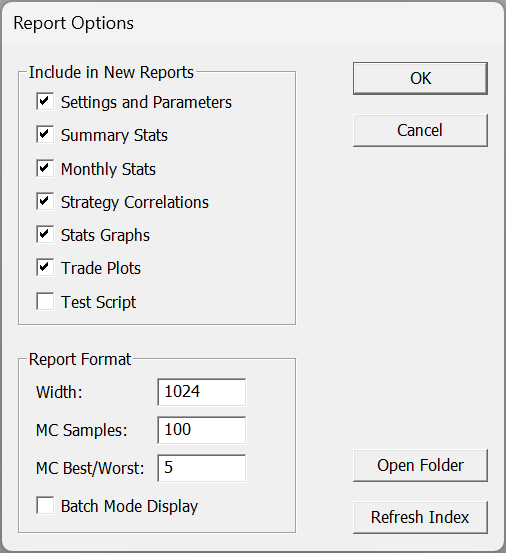Navigation: Backtest Engine Details > Asset Allocation and Position Sizing

RealTest makes it easy to specify any imaginable asset allocation and position sizing model.

Each Strategy definition can optionally (and should usually) include two key elements: Allocation and Quantity.

The formulas that you provide for Allocation and Quantity can use syntax elements such as S.StartEquity, S.Equity, S.Alloc, and the Combined() and Extern() functions to govern both the size of the "virtual account" used by the strategy and the size of each position taken.

Using these syntax elements in these formulas makes it possible to:

allocate different fractions of the initial account balance to different strategies

keep each strategy to its own equity pool, or let multiple strategies share a larger pool or the entire account

periodically re-balance the allocation of a strategy relative to other strategies in a shared pool

control how often (if ever) to compound the equity used by a strategy

change the allocation or position size for a strategy based on the recent results of one or more other strategies

To make the best use of these capabilities, it is useful to understand how the backtest engine uses the Allocation and Quantity formulas.

When a test begins, the following things happen:

1.The the value of the AccountSize setting is assigned to both S.StartEquity and S.Equity for each strategy, and for the Combined statistics.

2.If there is no Allocation formula, this same value is also assigned to S.Alloc for each strategy as a default initial allocation.

3.If there is an Allocation formula, then it is evaluated and the result becomes the initial allocation.

As a test runs, the following things occur on each virtual day of the simulation:

1.At the start of each trading day, before any other formulas are evaluated, Allocation is recalculated for each strategy. The result can be accessed in any other formula using S.Alloc. (Like all stat elements, S.Alloc is a time-series array, so prior values can also be accessed using offset syntax, e.g. S.Alloc, a moving average can be applied if desired, etc.)

2.Before a position is entered, the Quantity formula is evaluated to determine its position size (quantity of shares or contracts). Typically, the Quantity calculation will be based on S.Alloc. For example, S.Alloc / 10 / FillPrice would size the position to approximately 10% of current allocation for this strategy. (Quantity is always rounded to the nearest whole share.)

3.As each trade is exited, all of the relevant stats elements are updated, including S.Equity.

4.At the end of each trading day, all of the tracking stats (minimum and maximum equity, current and maximum drawdown, etc.) are updated.

Note that there is no reason that you need to use Allocation and S.Alloc. For a single-strategy system, you can simply specify Quantity based on S.Equity. Even for a multi-strategy system, it's fine to specify a fraction of S.Equity in the Quantity formula for each strategy rather than adding the Allocation layer. (If there is no Allocation formula then S.Alloc is always the same as S.Equity).

The main reason to use the Allocation layer is to specify how you want a common equity pool shared among multiple strategies. A multi-strategy backtest run without an Allocation formula will simply keep each strategy's capital separate, as if they were being run in different accounts. To model a scenario where strategies share a common equity pool, use:The initial allocation value of a strategy becomes the basis of its test statistics.

For example, consider an account that gains 20% in a year by running two strategies that each use half the account and perform equally well. Both strategies allow up to 5 open positions and size each position to 20% of their allocation.

This could be expressed two ways in the script:orEither way, the set of simulated trades and the combined statistics for the test will be identical.

The only difference will be in the individual strategy statistics. (To see individual strategy statistics in a multi-strategy backtest, use the Test Summary Report feature.)

In the first example, each strategy would show a 10% return, while in the second example, each would show a 20% return.

The choice of which approach to use is therefore a matter of personal preference.

Copyright © 2020-2021 Systematic Solutions, LLC